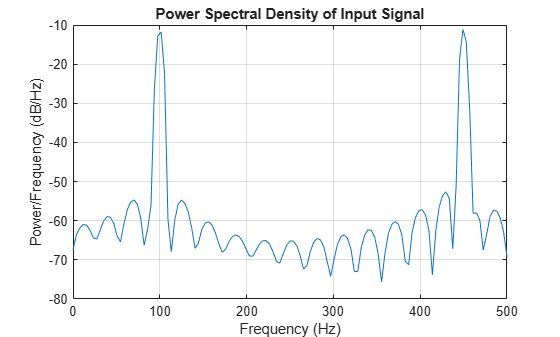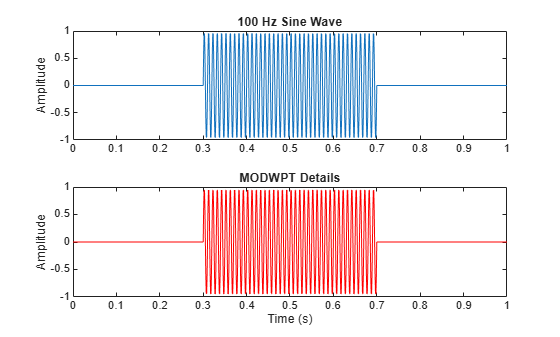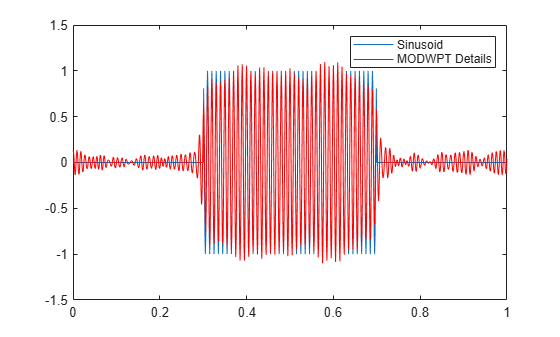modwptdetails

Maximal overlap discrete wavelet packet transform details

Description

example

w = modwptdetails(x) returns the maximal overlap discrete wavelet packet transform (MODWPT) details for the 1-D real-valued signal, x. The MODWPT details provide zero-phase filtering of the signal. By default, modwptdetails returns only the terminal nodes, which are at level 4 or at level floor(log2(numel(x))), whichever is smaller.

Note

To decide whether to use modwptdetails or modwpt, consider the type of data analysis you need to perform. For applications that require time alignment, such as nonparametric regression analysis, use modwptdetails. For applications where you want to analyze the energy levels in different packets, use modwpt. For more information, see Algorithms.

example

w = modwptdetails(x,wname) uses the orthogonal wavelet filter specified by wname.

example

w = modwptdetails(x,lo,hi) uses the orthogonal scaling filter, lo, and wavelet filter, hi.

w = modwptdetails(___,lev) returns the terminal nodes of the wavelet packet tree at positive integer level lev.

example

[w,packetlevs] = modwptdetails(___) returns a vector of transform levels corresponding to the rows of w.

[w,packetlevs,cfreq] = modwptdetails(___) returns cfreq, the center frequencies of the approximate passbands corresponding to the MODWPT details in w.

example

[___] = modwptdetails(___,'FullTree',tf), where tf is false, returns details about only the terminal (final-level) wavelet packet nodes. If you specify true, then modwptdetails returns details about the full wavelet packet tree down to the default or specified level. The default for tf is false.

Examples

collapse all

Obtain the MODWPT of an electrocardiogram (ECG) signal using the default length 18 Fejer-Korovkin ('fk18') wavelet and the default level, 4.

wptdetails = modwptdetails(wecg);

Demonstrate that summing the MODWPT details over each sample reconstructs the signal. The largest absolute difference between the original signal and the reconstruction is on the order of $1{0}^{-11}$, which demonstrates perfect reconstruction.

xrec = sum(wptdetails);
max(abs(wecg-xrec'))
ans = 1.7903e-11

Obtain the MODWPT details for a signal containing 100 Hz and 450 Hz sine waves. Each row of the modwptdetails output corresponds to a separate frequency band.

dt = 0.001;
fs = 1/dt;
t = 0:dt:1;
x = (sin(2*pi*100*t)+sin(2*pi*450*t));
[lo,hi] = wfilters('fk22');
wptdetails = modwptdetails(x,lo,hi);

Use modwpt to obtain the energy and center frequencies of the signal. Plot the energy in the wavelet packets. The fourth and fifteenth frequency bands contain most of the energy. Other frequency bands have significantly less energy. The frequency ranges of fourth and fifteenth bands are approximately 94-125 Hz and 438-469 Hz, respectively.

[wpt,~,cfreqs,energy] = modwpt(x,lo,hi);
figure
bar(1:16,energy);
xlabel('Packet')
ylabel('Packet Energy')
title('Energy by Wavelet Packet')Plot the power spectral density of the input signal.

pwelch(x,[],[],[],fs,'onesided');
title('Power Spectral Density of Input Signal')Show that the MODWPT details have zero-phase shift from the 100 Hz input sine.

p4 = wptdetails(4,:);
plot(t,sin(2*pi*100*t).*(t>0.3 & t<0.7))
hold on
plot(t,p4.*(t>0.3 & t<0.7),'r')
legend('Sine Wave','MODWPT Details')Obtain the MODWPT details for a 100 Hz time-localized sine wave in noise. The sampling rate is 1000 Hz. Obtain the MODWPT at level 4 using the length 22 Fejer-Korovkin ('fk22') wavelet.

dt = 0.001;
t = 0:dt:1;
x = cos(2*pi*100*t).*(t>0.3 & t<0.7)+0.25*randn(size(t));
wptdetails = modwptdetails(x,'fk22');
p4 = wptdetails(4,:);

Plot the MODWPT details for level 4, packet number 4. The MODWPT details represent zero-phase filtering of the input signal with an approximate passband of $\left[3Fs/{2}^{5},4Fs/{2}^{5}\right)$, where $Fs$ is the sampling frequency.

plot(t,cos(2*pi*100*t).*(t>0.3 & t<0.7));
hold on
plot(t,p4,'r')
legend('Sine Wave','MODWPT Details')Obtain the MODWPT details of an ECG waveform using the length 18 Fejer-Korovkin scaling and wavelet filters.

[lo,hi] = wfilters('fk18');
wpt = modwptdetails(wecg,lo,hi);

Obtain the MODWPT details for the full wavelet packet tree of an ECG waveform. Use the default length 18 Fejer-Korovkin ('fk18') wavelet. Extract and plot the node coefficients at level 3, node 2.

[w,packetlevels] = modwptdetails(wecg,'FullTree',true);
p3 = w(packetlevels==3,:);
plot(p3(3,:))
title('Level 3, Node 2 MODWPT Details')Input Arguments

collapse all

Input signal, specified as a real-valued row or column vector. x must have at least two elements.

Data Types: double

Analyzing wavelet, specified as one of the following:

• 'haar' — Haar wavelet

• 'dbN' — Extremal phase Daubechies wavelet with N vanishing moments, where N is a positive integer from 1 to 45.

• 'symN' — Symlets wavelet with N vanishing moments, where N is a positive integer from 2 to 45.

• 'coifN' — Coiflets wavelet with N vanishing moments, where N is a positive integer from 1 to 5.

• 'fkN' — Fejér-Korovkin wavelet with N coefficients, where N = 4, 6, 8, 14, 18 and 22.

Scaling filter, specified as an even-length real-valued vector. lo must satisfy the conditions necessary to generate an orthogonal scaling function. You can specify the lo and hi scaling-wavelet filter pair only if you do not specify wname.

Wavelet filter, specified as an even-length real-valued vector. hi must satisfy the conditions necessary to generate an orthogonal wavelet. You can specify the lo and hi scaling-wavelet filter pair only if you do not specify wname.

Transform level, specified as a positive integer less than or equal to floor(log2(numel(x))).

Return tree option, specified as false or true. If tf is false, then modwptdetails returns details about only the terminal (final-level) wavelet packet nodes. If you specify true, then modwptdetails returns details about the full wavelet packet tree down to the default or specified level.

For the full wavelet packet tree, w is a 2j+1-2-by-numel(x) matrix. Each level j has 2j wavelet packet details.

Output Arguments

collapse all

Wavelet packet tree details, returned as a matrix with each row containing the sequency-ordered wavelet packet details for the terminal nodes. The terminal nodes are at level 4 or at level floor(log2(numel(x))), whichever is smaller. The MODWPT details are zero-phase-filtered projections of the signal onto the subspaces corresponding to the wavelet packet nodes. The sum of the MODWPT details over each sample reconstructs the original signal.

For the default terminal nodes, w is a 2j-by-numel(x) matrix. For the full packet table, at level j, w is a 2j+1-2-by-numel(x) matrix of sequency-ordered wavelet packet coefficients by level and index. The approximate passband for the nth row of w at level j is $\left[\frac{n-1}{{2}^{\left(j+1\right)}},\frac{n}{{2}^{\left(j+1\right)}}\right)$ cycles per sample, where n = 1,2,...,2j.

Transform levels, returned as a vector. The levels correspond to the rows of w. If w contains only the terminal level coefficients, packetlevs is a vector of constants equal to the terminal level. If w contains the full wavelet packet tree of details, packetlevs is a vector with 2j-1 elements for each level, j. To select all the MODWPT details at a particular level, use packetlevs with logical indexing.

Center frequencies of the approximate passbands in the w rows, returned as a vector. The center frequencies are in cycles per sample. To convert the units to cycles per unit time, multiply cfreq by the sampling frequency.

Algorithms

The MODWPT details (modwptdetails) are the result of zero-phase filtering of the signal. The features in the MODWPT details align exactly with features in the input signal. For a given level, summing the details for each sample returns the exact original signal.

The output of the MODWPT (modwpt) is time delayed compared to the input signal. Most filters used to obtain the MODWPT have a nonlinear phase response, which makes compensating for the time delay difficult. All orthogonal scaling and wavelet filters have this response, except the Haar wavelet. It is possible to time align the coefficients with the signal features, but the result is an approximation, not an exact alignment with the original signal. The MODWPT partitions the energy among the wavelet packets at each level. The sum of the energy over all the packets equals the total energy of the input signal.

 Percival, D. B., and A. T. Walden. Wavelet Methods for Time Series Analysis. Cambridge, UK: Cambridge University Press, 2000.

 Walden, A.T., and A. Contreras Cristan. “The phase-corrected undecimated discrete wavelet packet transform and its application to interpreting the timing of events.” Proceedings of the Royal Society of London A. Vol. 454, Issue 1976, 1998, pp. 2243-2266.

Wavelet Toolbox DocumentationGet trial now